# RS Aggarwal Class 7 Solutions Chapter 12 Simple Interest CCE Test Paper

In this chapter, we provide RS Aggarwal Solutions for Class 7 Chapter 12 Simple Interest CCE Test Paper for English medium students, Which will very helpful for every student in their exams. Students can download the latest RS Aggarwal Solutions for Class 7 Chapter 12 Simple Interest CCE Test Paper Maths pdf, free RS Aggarwal Solutions Class 7 Chapter 12 Simple Interest CCE Test Paper Maths book pdf download. Now you will get step by step solution to each question.

### RS Aggarwal Solutions for Class 7 Chapter 12 Simple Interest CCE Test Paper Download PDF

Question 1.
Solution:Question 2.
Solution:
Let the sum be ₹ xQuestion 3.
Solution:
P = ₹ 3625, A = ₹ 4495, T = 2 years
S.I. = A – P = ₹ 4495 – ₹ 3625 = ₹ 870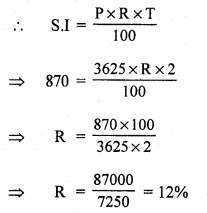Question 4.
Solution:
P = ₹ 3600, A = ₹ 4410, R = 9%
S.I. = A – P = ₹ 4410 – ₹ 3600 = ₹ 810Question 5.
Solution:
Let the sum be ₹ x
Amount = ₹ 2x
S.I. = (2x – x) = ₹ x
Time = 12 years
P = x, S.I. = x, T = 12 yearsQuestion 6.
Solution:
Let the sum be ₹ 4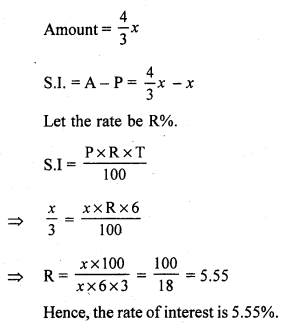Mark (✓) against the correct answer in each of the following :
Question 7.
Solution:
(c) 9%
Let the sum be ₹ x
A = 49×40
We know:
A = P + S.I.
S.I. = A – PQuestion 8.
Solution:
(c) ₹ 3500
A = ₹ 3626, R = 6%,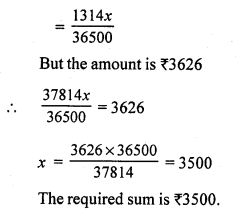Question 9.
Solution:
(a) 9 months
P = ₹ 6000, A = ₹ 6360
S.I. = A – P = 6300 – 6000 = ₹ 360Question 10.
Solution:
(c) 12%
Let the sum be ₹ x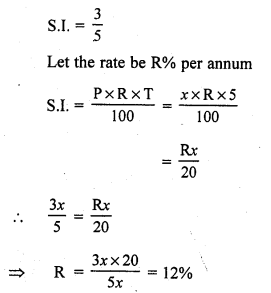Question 11.
Solution: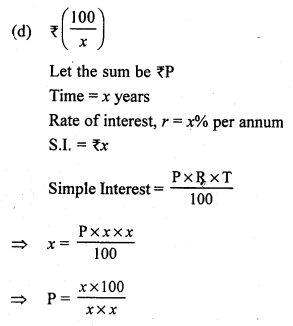P = ₹ 100x

Question 12.
Solution:
(b) 10%
Let the sum be ₹ x
Amount ₹ 2x
Time =10 years
S.I. = A – P = 2x – x = ₹ x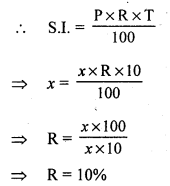Question 13.
Solution: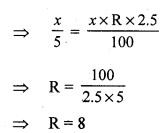Question 14.
Solution:
(i) FalseAll Chapter RS Aggarwal Solutions For Class 7 Maths

—————————————————————————–

All Subject NCERT Exemplar Problems Solutions For Class 7

All Subject NCERT Solutions For Class 7

*************************************************

I think you got complete solutions for this chapter. If You have any queries regarding this chapter, please comment on the below section our subject teacher will answer you. We tried our best to give complete solutions so you got good marks in your exam.

If these solutions have helped you, you can also share rsaggarwalsolutions.in to your friends.